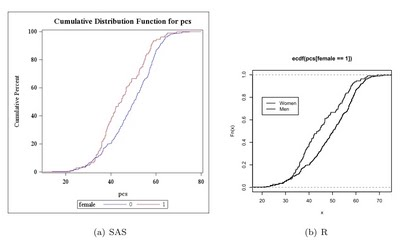Want to share your content on R-bloggers? click here if you have a blog, or here if you don't.

The empirical cumulative density function (CDF) (section 5.1.16) is a useful way to compare distributions between populations. The Kolmogorov-Smirnov (section 2.4.2) statistic D is the value of x with the maximum distance between the two curves. As an example, we compare the male and female distributions of pcs from the HELP data set described in the book. Here, we use built-in tools to plot the graph; in later entries we will build it from scratch for greater control.

We begin by reading in the data (section 1.1.14) as a comma separated file from the book web site (section 1.1.6).

SAS

```filename myurl
url 'http://www.math.smith.edu/sasr/datasets/help.csv'
lrecl=704;

proc import
datafile=myurl out=ds dbms=dlm;
delimiter=',';
getnames=yes;
run;
```

SAS proc univariate can do this plot automatically (section 5.1.15). It is designed to compare two groups within the data set, using the class statement (section 3.1.3).

```proc univariate data=ds;
var pcs;
class female;
cdfplot pcs / overlay;
run;
```

In R, the plot() function accepts ecdf() objects (section 5.1.15) as input. Applying this to pcs, conditional on including only the rows when female is 1 (section B.4.2) creates the first empirical CDF as well as the axes. The lines() function (section 5.2.1) also accepts ecdf() objects as input, and applying this to pcs when female is 0 adds the second empirical CDF to the existing plot. A legend (section 5.2.14) is added to show which curve is which. (Note that the Blogger software prevents displaying this image large enough to see the difference here, but it will be visible when run locally.

R

```> ds  attach(ds)
> plot(ecdf(pcs[female==1]), verticals=TRUE, pch=46)
> lines(ecdf(pcs[female==0]), verticals=TRUE, pch=46)
> legend(20, 0.8, legend=c("Women", "Men"), lwd=1:3)
```

Click the graphic below for a more legible image of the output.